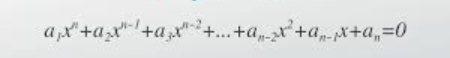Polynomial Solver Project (PreCal PreAP)The objective of this project is to use to solve a polynomial equation using algebra and trigonometry using a Microsoft PowerPoint presentation or Prezi Presentation.

Students will complete the project based on the individual or group assignments provided by the teacher.

Individual and group assignments are now available:

*All students are responsible for submitting the project via email regardless if you are working alone or with a partner. If you are assigned a partner and unable to reach them, you must document in the submission email the attempts you done to get in contact with the partner.

(25 points) Student A will solve the polynomial equation by algebraic methods. The student must address the following to find all the roots of the equation.

• The number of real roots and complex roots using the Fundamental Theorem of Algebra
• The number of real positive roots and real negative roots using Descartes’ Rule of Signs
• The complete factorization of the polynomial equation with linear or irreducible factors using the Factor Theorem
• The real and complex roots of the polynomial equation using factoring and the Quadratic Formula

(25 points) Student B will solve the polynomial equation by using trigonometry. The student must address the following to find all the roots of the equation.

• The number of real roots and complex roots using the Fundamental Theorem of Algebra
• The trigonometric form of the real or complex number from the polynomial equation where θ is written in radians
• The real and complex roots of the polynomial equation using the nth-roots of a complex number formula.
• The complete factorization of the polynomial equation using the roots.

(50 points) Both students must collaborate and compile a complete Microsoft PowerPoint presentation or Prezi presentation. Your presentation must include the following:

• Name of each student, Class Period / Day
• Polynomial equation solved using algebra with work shown by Student A.
• Polynomial equation solved using trigonometry with work shown by Student B.